Question

# What is the probability of getting 3 sevens in a row on 3 independent rolls of...

What is the probability of getting 3 sevens in a row on 3 independent rolls of a pair of fair dice, given that the first two rolls were sevens? Provide your answer as a reduced fraction.

Probability = Favorable Outcomes / Total Outcomes

There are 6 sides on a dice. Therefore Probability of getting a 7 = 1 / 6

Given that we have got a seven in the first 2 rolls. Which means these events have occurred, and therefore the probability of each event = 1 * 1 = 1

Therefore the probability of a 7 coming on the 3rd roll, given that you already have a 7 in the first 2 rolls

P = 1 * 1 * (1 / 6) = 1 / 6

#### Earn Coins

Coins can be redeemed for fabulous gifts.

Similar Homework Help Questions
• ### A pair of dice is rolled. What is the probability of getting a sum of 2?...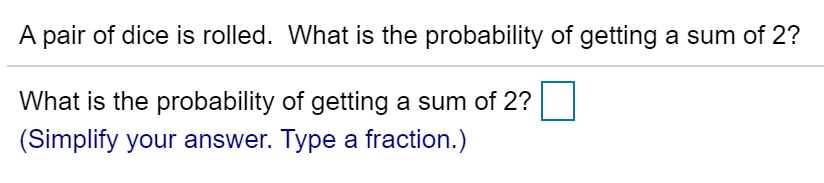A pair of dice is rolled. What is the probability of getting a sum of 2? What is the probability of getting a sum of 2? (Simplify your answer. Type a fraction.)

• ### You roll two fair dice, a green one and a red one. (a) What is the probability of getting a sum of 7? (Enter your answ...

You roll two fair dice, a green one and a red one. (a) What is the probability of getting a sum of 7? (Enter your answer as a fraction.) 1 (b) What is the probability of getting a sum of 11? (Enter your answer as a fraction.) 2 (c) What is the probability of getting a sum of 7 or 11? (Enter your answer as a fraction.) Are these outcomes mutually exclusive? Yes No

• ### (A) If you roll 3 six-sided dice, what is the probability of getting an overall score...

(A) If you roll 3 six-sided dice, what is the probability of getting an overall score of 8? (B) If you roll 3 six-sided dice 4 times, what is the probability of getting an overall score of 8 four times in a row? (C) If you roll 3 six-sided dice 2 times, what is the probability of getting an overall score of 8 on the first roll and an overall score of 3 on the second roll?

• ### What is the probability of getting a sum of 3 when three standard dice are rolled

What is the probability of getting a sum of 3 when three standard dice are rolled? Express your answer as a common fraction.

• ### What is the probability of getting a total of 5 or 9 or 13 when a...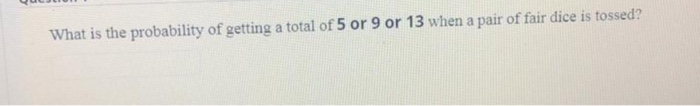What is the probability of getting a total of 5 or 9 or 13 when a pair of fair dice is tossed?

• ### 3. A pair of fair dice are tossed. Find the probability of getting (a) a total...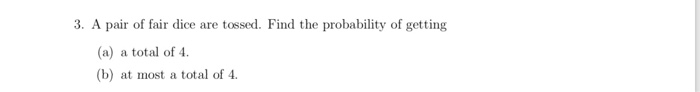3. A pair of fair dice are tossed. Find the probability of getting (a) a total of 4. (b) at most a total of 4

• ### Problem 2. (30 points) a) (5 points) In rolling 3 fair dice, what is the probability...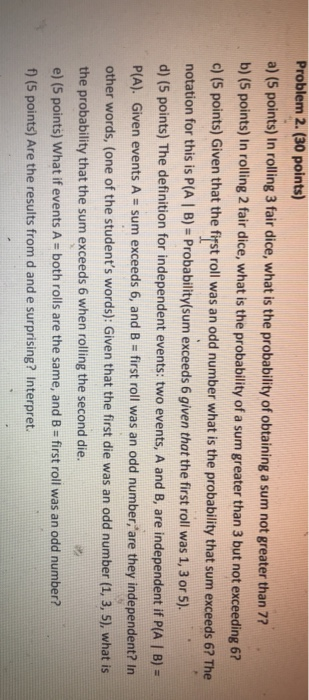Problem 2. (30 points) a) (5 points) In rolling 3 fair dice, what is the probability of obtaining a sum not greater than 77 b) (5 points) In rolling 2 fair dice, what is the probability of a sum greater than 3 but not exceeding 6? o) (5 points) Given that the frstroll was an odd number what is the probability that sum exceeds 6? The notation for this is P(A I B)- Probability(sum exceeds 6 given that the first...

• ### d. After 18 rolls, what would be the empirical probability of getting a three on at least one of those rolls? Also, list the likelihood scale term from the table above. Percent Probability Em...

d. After 18 rolls, what would be the empirical probability of getting a three on at least one of those rolls? Also, list the likelihood scale term from the table above. Percent Probability Empirical Probability (Rounded to the Nearest Whole Percent) (2 points) ? Likelihood Scale Term (2 points) ? Show your work: What do you notice about the answers for parts c and d above? (2 points) ? Roll the dice 18 times and keep track of what is...

• ### Conditional Probability Two fair dice are rolled: (a) Express the sample space S in set builder...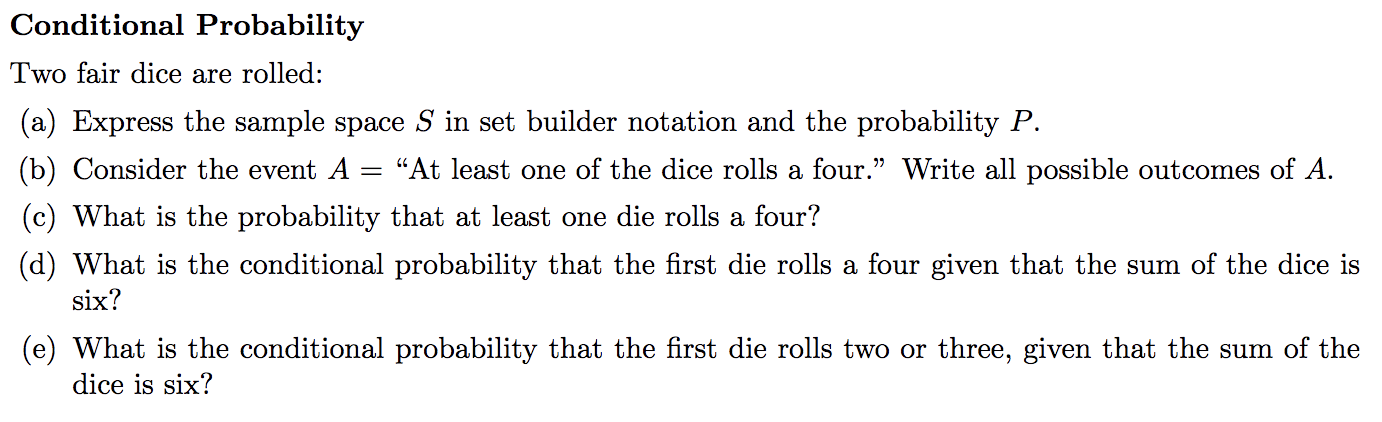Conditional Probability Two fair dice are rolled: (a) Express the sample space S in set builder notation and the probability P "At least one of the dice rolls a four." Write all possible outcomes of A (b) Consider the event A (c) What is the probability that at least one die rolls a four? (d) What is the conditional probability that the first die rolls a four given that the sum of the dice is six? (e) What is the...

• ### Four dice are rolled. (a) Find the probability of getting all sixes. (Enter your answer as...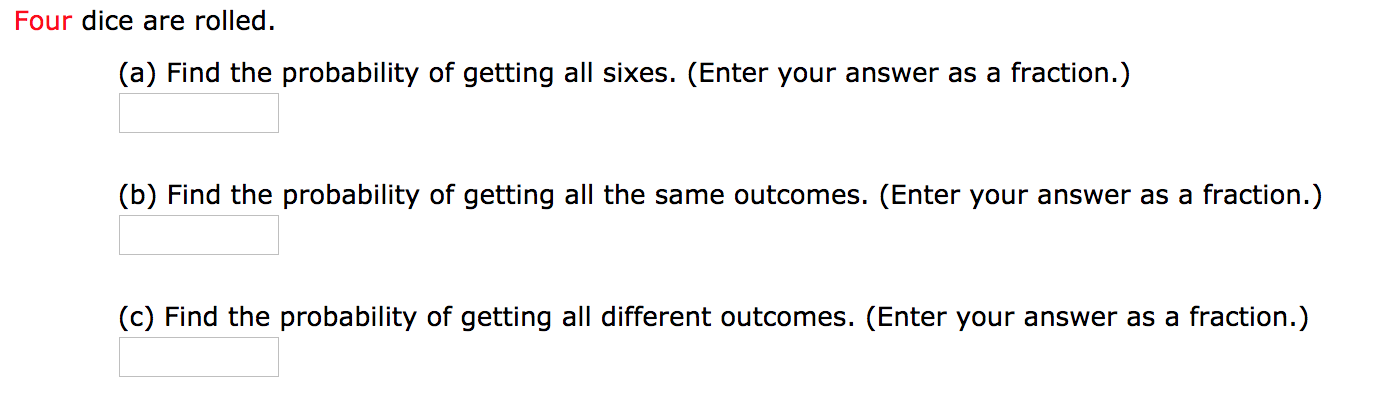Four dice are rolled. (a) Find the probability of getting all sixes. (Enter your answer as a fraction.) (b) Find the probability of getting all the same outcomes. (Enter your answer as a fraction.) (c) Find the probability of getting all different outcomes. (Enter your answer as a fraction.)